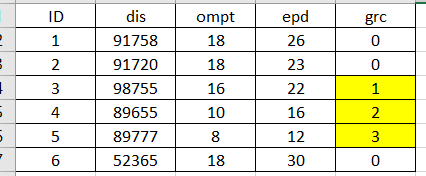# Loop query help

Hi Guys,

Please see below the test data and syntax. What I want to populate a one field based on case logic. I want to populate with 0 if CASE WHEN dis <> LAG(dis) over(ORDER BY dis) OR dis = '' or dis = '00000' or ompt > 16 or epd > 22 then '0' ELSE grab the previous value +1 Until it got 0 zero again.

Here is the end result that I am looking. Any advice would be highly appreciated.CREATE TABLE g_test(

id int IDENTITY(1,1),

dis varchar(10),

ompt int,

epd int,

Gra int

)

INSERT INTO g_test (dis,ompt,epd)

SELECT '91758',18,26

UNION ALL

SELECT '91720',18,23

UNION ALL

SELECT '98755',16,22

UNION ALL

SELECT '89655',10,16

UNION ALL

SELECT '89777',8,12

UNION ALL

SELECT '52365',18,30

SELECT

ID,

dis,

ompt,

epd,

CASE WHEN dis <> LAG(dis) over(ORDER BY dis) OR dis = '' or dis = '00000' or ompt > 16 or epd > 22 then '0'

else DENSE_RANK () over(partition by id ORDER BY id) end as grc

FROM g_test

Your logic does not make sense to me. The following produces the required result from the test data:

``````WITH Adds
AS
(
SELECT T.id, T.dis, T.ompt, T.epd, T.gra
,X.Plus1
,CASE
WHEN X.Plus1 <> LAG(X.Plus1) OVER (ORDER BY (T.id))
THEN 1
ELSE 0
END AS Boundary
FROM g_test T
CROSS APPLY
(
VALUES
(
CASE WHEN ompt > 16 OR epd > 22 THEN 0 ELSE 1 END
)
) X (Plus1)
)
,Grps
AS
(
SELECT id, dis, ompt, epd, gra, Plus1
,SUM(Boundary) OVER (ORDER BY id) AS Grp
)
SELECT id, dis, ompt, epd, gra
,CASE
WHEN Plus1 = 1
THEN ROW_NUMBER() OVER (PARTITION BY Grp ORDER BY id)
ELSE 0
END AS Result
FROM Grps;
/*
,Results
AS
(
SELECT id, gra
,CASE
WHEN Plus1 = 1
THEN ROW_NUMBER() OVER (PARTITION BY Grp ORDER BY id)
ELSE 0
END AS Result
FROM Grps
)
UPDATE Results
SET gra = Result;

select * from g_test;
*/``````

I want to say thank you very much! After I carefully reviewed our data and created a sample data. It looks like I need "gra" number based on the "dis" field. Same above logic. I created sample data below. However, the results are not correct. After you run below query you will see the row #4 "gra = 2". It should be 1. The sequence number should start 0,1,2,3... Please guide me what am I doing wrong.

Blockquote

DECLARE @g_test5 TABLE
(
id int IDENTITY(1,1),
dis varchar(10),
ompt int,
epd int,
Gra int

)

INSERT INTO @g_test5 (dis,ompt,epd)
SELECT '91758',18,26
UNION ALL
SELECT '91720',18,23
UNION ALL
SELECT '98755',16,22
UNION ALL
SELECT '98755',10,16
UNION ALL
SELECT '98755',8,12
UNION ALL
SELECT '98755',18,30

SELECT
ID,
dis,
ompt,
epd,
CASE WHEN dis <> LAG(dis) over(ORDER BY dis) OR dis = '' or dis = '00000' or ompt > 16 or epd > 22 then '0'
else ROW_NUMBER () over(partition by dis ORDER BY id) end as grc

FROM @g_test5

Blockquote

Please explain how the dis logic works with the first set of test data. (g_test)
Then give test data that combines g_test and g_test5.
Then show expected output.

Please ignore the g_test. @g_test5 is the correct sample dataset.
@g_test5 sample query and dataset already provided.
Let me know if the question is still not clear.
Thanks.

Sorry forgot to mention. The above code that you provided for g_test, works perfectly. Thank you for that. However, It is very hard to implement with the existing SP. So I went back and analyzed the data to use the ROW_NUMBER based on dis. However, the problem I am facing. The row_number starts with 2, instead of 1. If you run the above sample code (@g_test5), you will see what I am talking about. Appreciate your help!

It is still not clear what you want so this is my last guess:

``````WITH Adds
AS
(
SELECT T.id, T.dis, T.ompt, T.epd, T.gra
,X.Plus1
,CASE
WHEN X.Plus1 <> LAG(X.Plus1) OVER (ORDER BY T.dis, T.id)
THEN 1
ELSE 0
END AS Boundary
FROM @g_test5 T
CROSS APPLY
(
VALUES
(
CASE
WHEN T.ompt > 16 OR T.epd > 22 OR T.dis IN ('','00000')
THEN 0
ELSE 1
END
)
) X (Plus1)
)
,Grps
AS
(
SELECT id, dis, ompt, epd, gra, Plus1
,SUM(Boundary) OVER (ORDER BY dis, id) AS Grp